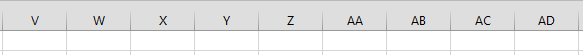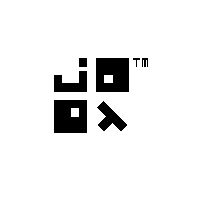# How to use Java 8 Functional Programming to Generate an Alphabetic Sequence

I’ve stumbled upon an interesting Stack Overflow question by user “mip”. The question was:
I’m looking for a way of generating an alphabetic sequence:
`A, B, C, ..., Z, AA, AB, AC, ..., ZZ.`
This can be quickly recognised as the headings of an Excel spreadsheet, which does precisely that:.So far, none of the answers employed any Java 8 functional programming, which I accepted as a challenge. We’re going to use jOOλ, because the Java 8 Stream API does not offer enough functionality for this task. (I stand corrected – thank you Sebastian, for this interesting answer) But first, let’s decompose the algorithm in a functional way. What we need are these components:
1. A (reproducible) representation of the alphabet
2. An upper bound, i.e. how many letters we want to produce. The requested sequence goes to `ZZ`, which means the upper bound would be 2
3. A way to combine each letter of the alphabet with the previously generated combined letters in a cartesian product
Let’s look into some code:

### 1. Generating the alphabet

We could be writing the alphabet like this:
```
List<String> alphabet = Arrays.asList("A", "B", ..., "Z");

```
but that would be lame. Let’s generate it instead, using jOOλ:
```
List<String> alphabet = Seq
.rangeClosed('A', 'Z')
.map(Object::toString)
.toList();

```
The above generates a “closed” range (Java-8-Stream-speak for a range with inclusive upper bound) of characters between `A` and `Z`, maps characters to strings and collects them into a list. So far so good. Now:

### 2. Using an upper bound

The requested sequence of characters includes:
```A .. Z, AA, AB, .. ZZ
```
But we could easily imagine to extend this requirement generally to produce the following, or even more.
```A .. Z, AA, AB, .. ZZ, AAA, AAB, .. ZZZ
```
For this, we’ll use again `rangeClosed()`:
```
// 1 = A .. Z, 2 = AA .. ZZ, 3 = AAA .. ZZZ
Seq.rangeClosed(1, 2)
.flatMap(length -> ...)
.forEach(System.out::println);

```
The idea here is to produce a new stream for each individual length in the range `[1 .. 2]`, and to flatten those streams into one single stream. `flatMap()` is essentially the same as a nested loop in imperative programming.

### 3. Combine letters in a cartesian product

This is the trickiest part: We need to combine each letter with each letter `length` times. For this, we’ll use the following stream:
```
Seq.rangeClosed(1, length - 1)
.foldLeft(Seq.seq(alphabet), (s, i) ->
s.crossJoin(Seq.seq(alphabet))
.map(t -> t.v1 + t.v2))
);

```
We’re using again `rangeClosed()` to produce values in the range `[1 .. length-1]`. `foldLeft()` is the same as `reduce()`, except that `foldLeft()` is guaranteed to go from “left to right” in a stream, without requiring the folding function to be associative. Whew. In other, more understandable words: `foldLeft()` is nothing else but an imperative loop. The “seed” of the loop, i.e. the loop’s initial value, is a complete alphabet (`Seq.seq(alphabet)`). Now, for every value in the range `[1 .. length-1]`, we produce a cartesian product (`crossJoin()`) between the letters “folded” so far and a new alphabet, and we concatenate each combination into a single new string (`t.v1` and `t.v2`). That’s it!

### Combining everything

The following simple program prints all the values from `A .. Z, AA .. ZZ, AAA .. ZZZ` to the console:
```
import java.util.List;

import org.jooq.lambda.Seq;

public class Test {
public static void main(String[] args) {
int max = 3;

List<String> alphabet = Seq
.rangeClosed('A', 'Z')
.map(Object::toString)
.toList();

Seq.rangeClosed(1, max)
.flatMap(length ->
Seq.rangeClosed(1, length - 1)
.foldLeft(Seq.seq(alphabet), (s, i) ->
s.crossJoin(Seq.seq(alphabet))
.map(t -> t.v1 + t.v2)))
.forEach(System.out::println);
}
}

```

### Disclaimer

This is certainly not the most optimal algorithm for this particular case. One of the best implementations has been given by an unnamed user on Stack Overflow:
```
import static java.lang.Math.*;

private static String getString(int n) {
char[] buf = new char[(int) floor(log(25 * (n + 1)) / log(26))];
for (int i = buf.length - 1; i >= 0; i--) {
n--;
buf[i] = (char) ('A' + n % 26);
n /= 26;
}
return new String(buf);
}

```
Unnecessary to say that the latter runs much much faster than the previous functional algorithm.

## 5 thoughts on “How to use Java 8 Functional Programming to Generate an Alphabetic Sequence”

1. Sebastian Millies says:

Why do you say that the Java 8 Stream API does not offer enough functionality for this task? In fact, it does.

I describe a pure Java 8 solution, with some explanations, at http://sebastian-millies.blogspot.de/2015/09/cartesian-products-with-kleisli.html“.

I am sure that solution is basically equivalent to your code, but it does not utilize jOOλ and I think brings out the structure of the problem rather better. Also, it would parallelize.

— Sebastian

1. Hi Sebastian,

Yes, it was me :-)
Very very nice, thank you very much for this writeup. I stand correctend and I’ll study your insights. Keep it up,

Lukas

2. What I like in Kotlin that tasks like that can be really easy:
val letters = ‘a’..’z’
letters flatMap { letters map { char -> it.toString() + char }

1. Would you mind explaining? There seems to be a lot of implicit stuff there. Such as, what’s `char` and what’s `it`?

3. danieldietrich says:

I’m a little late to the party but for the sake of completeness… using Javaslang we can do it like so in Java 8:

```Stream Σ =
Stream.rangeClosed('A', 'Z').map(String::valueOf);

Σ.appendSelf(s -> s.crossProduct(Σ).map(t -> t._1 + t._2))
.take(max).stdout();
```